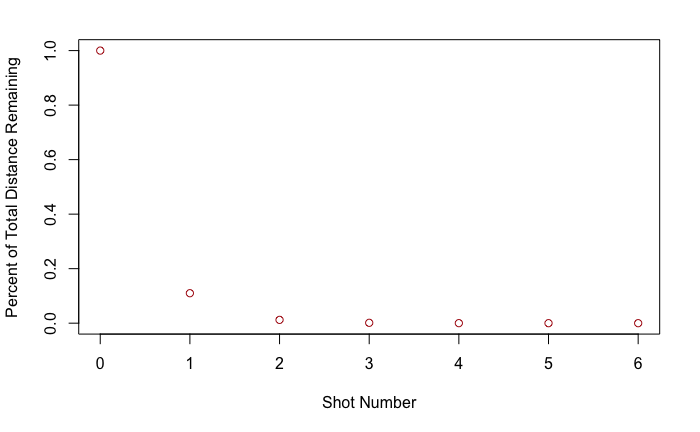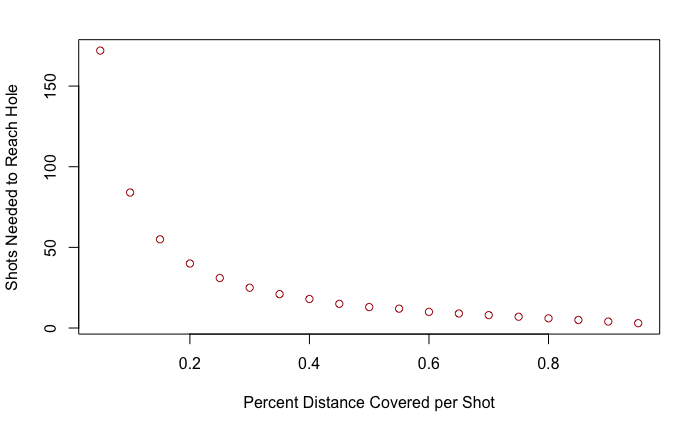# Fivethirtyeight Riddler: Golf Percentages

This week’s Riddler Express was one I managed to solve analytically, but I’ve also included some plots in R for some post-problem analysis, just for fun.

# Riddler Express

From Dan Levin comes a hazardous riddle for the ironclad and eagle-eyed:

The U.S. Open concluded last weekend, with physics major Bryson DeChambeau emerging victorious. Seeing his favorite golfer win his first major got Dan thinking about the precision needed to be a professional at the sport.

A typical hole is about 400 yards long, while the cup measures a mere 4.25 inches in diameter. Suppose that, with every swing, you hit the ball X percent closer to the center of the hole. For example, if X were 75 percent, then with every swing the ball would be four times closer to the hole than it was previously.

For a 400-yard hole, assuming there are no hazards (water, sand or otherwise) in the way, what is the minimum value of X so that you’ll shoot par, meaning you’ll hit the ball into the cup in exactly four strokes?

## Solution

Let the initial distance from the tee to the hole be $$d_0$$. The percent of the total distance covered by each shot is given by $$x$$. Then, the remaining distance to the hole after the first hit is given by

\begin{equation} d_1 = d_0 (1-x) \end{equation}

Similarly, the distance after the second hit is given by

\begin{equation} d_2 = d_1(1-x)
d_2 = (d_0(1-x))(1-x) = d_0(1-x)^2 \end{equation}

It follows that after $N$ strokes, the remaining distance is given by

\begin{equation} d_N = d_0(1-x)^N \end{equation}

To estimate when the hit would land in the hole, we want $$d_N=r$$, the hole’s radius. At this point, the center of the ball will lie on the edge of the hole and we can assume gravity will do the trick. If we want that to achieve a par of 4, then this must occur when $$N=4$$. The problem gives a course length of $$d_0=14,400$$ (400 yards in inches), and $$r=4.25/2=2.125$$.

\begin{equation} d_4 = d_0(1-x)^4
2.125 = 14400(1-x)^4
x \approx 0.89 \end{equation}

### Plots

Just to get a more visual understanding of the problem, I’m including some additional plots.

First, looking at percentage of distance covered as a function of shots for a “par-level” player (covering 89% of the distance per shot),Next, the number of strokes to make it in a hole at 400 yards for various distance percentage values. Solving for N gives,

\begin{equation} \frac{14400}{2.125} = (1-x)^N
N = \frac{\ln(14400/2.125)}{\ln(1-x)} \end{equation}

Of course, N is constrained to $$\Bbb Z^+$$ (positive integers), so a ceiling round is applied.## Accessing Public Baseball Data in Julia

Interoperability is the name of the game

I got a job!

## Box Score Thoughts: Tempering Run Expectations from Hits

Revisiting some old work, and handling some heteroscadasticity

## Gauging Home-field Advantage in 2020

Using a Bayesian GLM in order to see if a lack of fans translates to a lack of home-field advantage

## Fivethirtyeight Riddler: Golf Percentages

An analytical solution plus some plots in R (yes, you read that right, R)

## Fivethirtyeight Riddler: Astrophysical Signals

okay… I made a small mistake

## Classifying MLB Hit Outcomes - Part 4: Application and Reflection

Creating a practical application for the hit classifier (along with some reflections on the model development)

## Classifying MLB Hit Outcomes - Part 3: Studying Re-sampling Methods

Diving into resampling to sort out a very imbalanced class problem

## Fivethirtyeight Riddler: Can You Find The Fish In State Names?

Or, ‘how I learned the word pneumonoultramicroscopicsilicovolcanoconiosis’

## Classifying MLB Hit Outcomes - Part 2: Optimization

Amping up the hit outcome model with feature engineering and hyperparameter optimization

## Classifying MLB Hit Outcomes - Part 1: Model Selection

Can we classify the outcome of a baseball hit based on the hit kinematics?

## Applying to Work in MLB Front Offices - My Experience

A summary of my experience applying to work in MLB Front Offices over the 2019-2020 offseason

## Fivethirtyeight Riddler: How Low Can You Roll?

Busting out the trusty random number generator

## Astros 2017 K% Change

Perhaps we’re being a bit hyperbolic

## Fivethirtyeight Riddler: Which Baseball Team Will Win The Riddler Fall Classic?

Revisiting more fake-baseball for 538

## Evaluating Lance Lynn’s Unexpected 2019

A deep-dive into Lance Lynn’s recent dominance

## 2015-2016 Di-Higgs Combination

Fresh-off-the-press Higgs results!

## Fivethirtyeight Riddler: Can The Riddler Bros. Beat Joe DiMaggio’s Hitting Streak?

How do theoretical players stack up against Joe Dimaggio?

## Pheno2019 - ATLAS Searches for VH/HH Resonances

I went to Pittsburgh to talk Higgs

## Fivethirtyeight Riddler: Can You Turn America’s Pastime Into A Game Of Yahtzee?

If baseball isn’t random enough, let’s make it into a dice game

## US LHC Users Association 2018 - Why do we care about di-Higgs Production?

Or: how to summarize a PhD’s worth of work in 8 minutes

## Double Higgs Production at Colliders Workshop - VBF HH Generation and Benchmarks

Double the Higgs, double the fun!

## 2018 Reddit /r/Baseball Trade Deadline Game Statistics

A data-driven summary of the 2018 Reddit /r/Baseball Trade Deadline Game